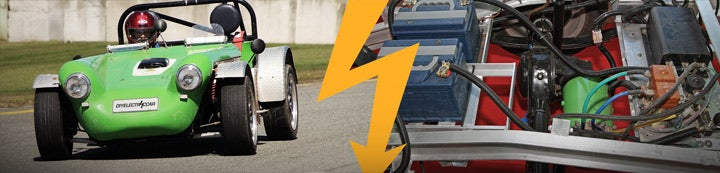1 - 2 of 2 Posts

#### EVDL List

·
##### Registered
Joined
·
70 Posts
Discussion Starter · ·
*Sometimes a little humor sets us up for a good weekend...
*

*
*

*We all love Bud Abbott and Lou Costello and their humorous routines like
=93Who =91s on First.=94*

*If Vaudeville were as popular today, and Lou bought an Electric Car from
Bud the next day this might happen;*

*a
*

*Bud; Hello, Electric Auto Emporium.*

*Lou; That car I bought from you doesn=92t start.*

*Bud; Did you charge the battery?*

*Lou; Yes, but it doesn=92t start.*

*Bud; Did you turn on the key?*

*Lou; Yes, but it doesn=92t start.*

*Bud; Did you turn the key to start and release it?*

*Lou; Yes, but the motor isn=92t starting.*

*Bud; Did you put it in gear?*

*Lou; No, it isn=92t starting.*

*Bud; Did you unplug the charging cable from the car?*

*Lou; I=92ll go look.*

*=3D=3D=3D=3D=3D20 minutes later=3D=3D=3D=3D=3D*

*Bud; Hello, Electric Auto Emporium. *

*Lou; It still won=92t start.*

*Bud, Did you turn on the key?*

*Lou, Yes, you better send me a Mechanic.*

*Bud; OK*

*=3D=3D=3D=3D=3DA few hours later=3D=3D=3D*

*Bud; Hello, Electric Auto Emporium. *

*Lou; It still won=92t start.*

*Bud; What did the mechanic do?*

*Lou; He climbed in and drove it around the block, then he left.*

*Bud; So everything is OK?*

*Lou; No, it still won=92t start.*

*Bud; Did you turn on the key and turn it to start?*

*Lou; Yes but it doesn=92t start*

*Bud; Can you put it in gear and press the accelerator?*

*Lou; No, I don=92t want to flood it and it isn=92t running.*

*Bud; What do you want me to do Sir? (Exasperated)*

*Lou; Come pick up this =93LEMON=94 and refund my money.*

*Bud; Did you read the driver=92s manual?*

*Lou; No, I know how to drive, I have been driving for 30 years.*

*Bud; Electric?*

*Lou; Yes this car is Electric.*

*Bud; Have you driven an Electric?*

*Lou; No, the DARN thing won=92t START!*
=3D=3D=3D=3D=3D=3D=3D=3D=3D=3D=3D=3D=3D=3D=3D=3D=3D=3D=3D=3D=3D=3D=3D=3D=3D=
=3D=3D=3D=3D=3D=3D=3D=3D=3D=3D=3D=3D=3D=3D=3D=3D=3D=3D=3D=3D=3D=3D=3D=3D=3D=
=3D=3D=3D=3D=3D=3D=3D=3D=3D=3D=3D=3D=3D

-- =

Regards,
*Dennis Lee Miles* (Director) *E.V.T.I. inc*.
EVTI-EVAEducation Chapter
*
Phone (813) ID4 - E V T I or (813) 434 - 3884 (I think word phone
numbers can be fun and good mnemonics aid memory.)
-------------- next part --------------
An HTML attachment was scrubbed...
URL: http://lists.sjsu.edu/mailman/private/ev/attachments/20101029/1ebe63c4=
/attachment.html =

_______________________________________________
| Multiple-address or CCed messages may be rejected.
| UNSUBSCRIBE: http://www.evdl.org/help/index.html#usub
| OTHER HELP: http://evdl.org/help/
| OPTIONS: http://lists.sjsu.edu/mailman/listinfo/ev

#### EVDL List

·
##### Registered
Joined
·
70 Posts
Discussion Starter · ·
Dennis,

I found this very funny. It happened to me, twice, but in reverse. I had =

become so accustomed to driving an EV that when my supervisor asked me to =

take her to the airport in her car and then park it in our office parking =

lot I got in, clicked the key to on as I would in my own cars, looked at he=
r =

and said "Your car won't start." I had forgotten you have to turn it more, =

until the starter kicks in. Then I did the same thing with an ICE I rented=
, =

even called the rental company to tell them the car wouldn't start. After =
a =

little while I remembered what the problem might be, got the car started an=
d =

had to call them back and try to explain that I was not a nut case and not =

to send anyone to help me.

Gail

----- Original Message ----- =

From: "Dennis Miles" <[email protected]>
To: "Electric Vehicle Discussion List" <[email protected]>
Sent: Thursday, October 28, 2010 10:37 PM
Subject: [EVDL] A little humor for Friday...(It is about an electric car !)

*Sometimes a little humor sets us up for a good weekend...
*

*
*

*We all love Bud Abbott and Lou Costello and their humorous routines like
=93Who =91s on First.=94*

*If Vaudeville were as popular today, and Lou bought an Electric Car from
Bud the next day this might happen;*

*a
*

*Bud; Hello, Electric Auto Emporium.*

*Lou; That car I bought from you doesn=92t start.*

*Bud; Did you charge the battery?*

*Lou; Yes, but it doesn=92t start.*

*Bud; Did you turn on the key?*

*Lou; Yes, but it doesn=92t start.*

*Bud; Did you turn the key to start and release it?*

*Lou; Yes, but the motor isn=92t starting.*

*Bud; Did you put it in gear?*

*Lou; No, it isn=92t starting.*

*Bud; Did you unplug the charging cable from the car?*

*Lou; I=92ll go look.*

*=3D=3D=3D=3D=3D20 minutes later=3D=3D=3D=3D=3D*

*Bud; Hello, Electric Auto Emporium. *

*Lou; It still won=92t start.*

*Bud, Did you turn on the key?*

*Lou, Yes, you better send me a Mechanic.*

*Bud; OK*

*=3D=3D=3D=3D=3DA few hours later=3D=3D=3D*

*Bud; Hello, Electric Auto Emporium. *

*Lou; It still won=92t start.*

*Bud; What did the mechanic do?*

*Lou; He climbed in and drove it around the block, then he left.*

*Bud; So everything is OK?*

*Lou; No, it still won=92t start.*

*Bud; Did you turn on the key and turn it to start?*

*Lou; Yes but it doesn=92t start*

*Bud; Can you put it in gear and press the accelerator?*

*Lou; No, I don=92t want to flood it and it isn=92t running.*

*Bud; What do you want me to do Sir? (Exasperated)*

*Lou; Come pick up this =93LEMON=94 and refund my money.*

*Bud; Did you read the driver=92s manual?*

*Lou; No, I know how to drive, I have been driving for 30 years.*

*Bud; Electric?*

*Lou; Yes this car is Electric.*

*Bud; Have you driven an Electric?*

*Lou; No, the DARN thing won=92t START!*
=3D=3D=3D=3D=3D=3D=3D=3D=3D=3D=3D=3D=3D=3D=3D=3D=3D=3D=3D=3D=3D=3D=3D=3D=3D=
=3D=3D=3D=3D=3D=3D=3D=3D=3D=3D=3D=3D=3D=3D=3D=3D=3D=3D=3D=3D=3D=3D=3D=3D=3D=
=3D=3D=3D=3D=3D=3D=3D=3D=3D=3D=3D=3D=3D

_______________________________________________